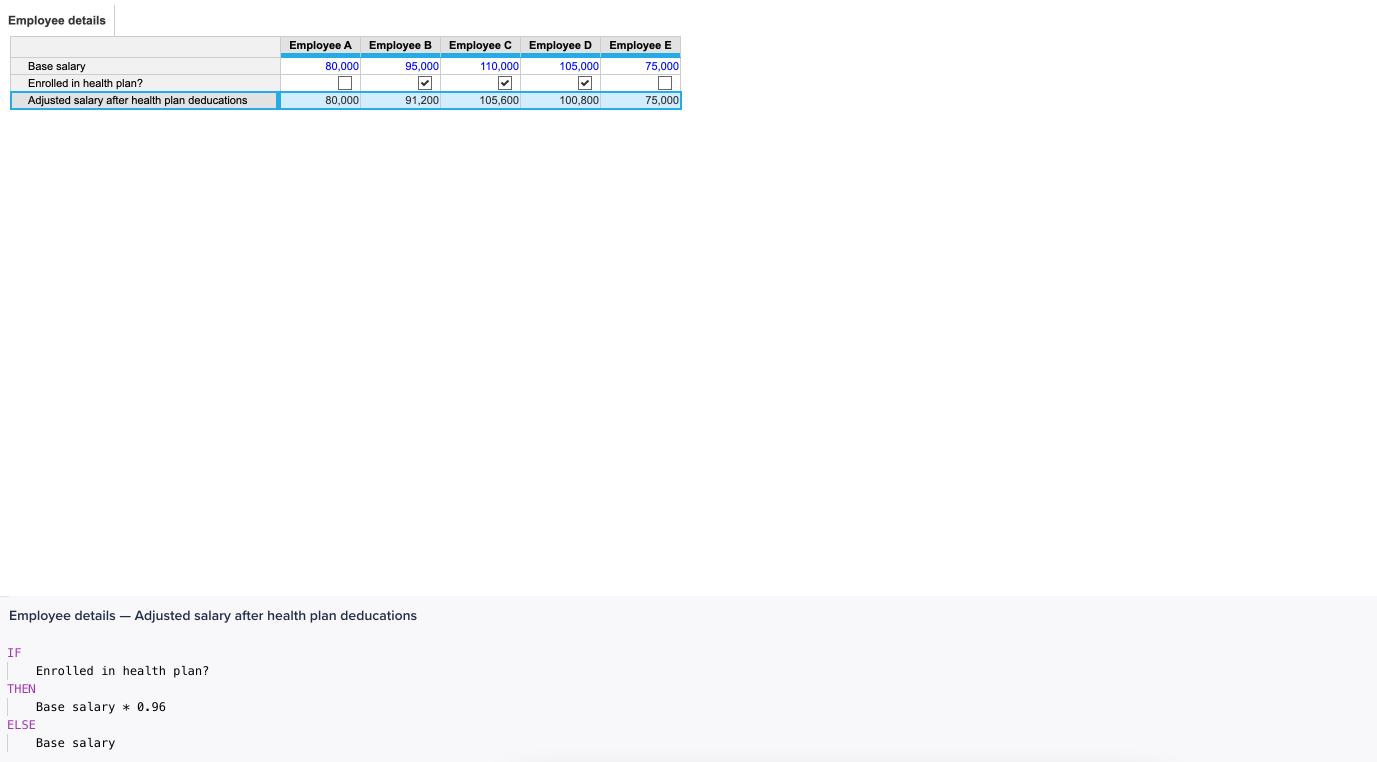Add formulas with the formula editor, especially when you write complex formulas. The editor uses syntax highlighting and checking to help you read and correct the code. Autocomplete and smart formatting help you accurately reference your functions, and automatically format your formula. You can also switch between modules while writing formulas.

Note: Always format the line item before entering a formula in it. You can only submit a formula if the result matches the format of the cell. For example, if the formula results in a date, the line item must have a date format.

When you write formulas, ensure that you follow these requirements:

• Close open parentheses.
• Enter all required arguments for any functions that you use.
• Do not nest more than 100 functions in a single formula.
• Enclose line item names that contain hyphens, numbers, or operators (+ - / *) with single quotation marks.

To find information on functions, operators, and how to write formulas, see All functions.

To add a formula to a line item using the formula editor:

1. Select Blueprint , then select the line item. In the formula bar, the line item name appears next to the editing field.
2. In the formula bar, select the line item name, or Expand to the left of the line item name to open the formula editor in preview mode.
Note: Valid formulas that contain IF THEN ELSE functions are indented in the formula editor.1. To activate the formula editor:
• Select Edit .
• Double-click in the formula editor.
• Type in the formula editor.
2. Enter the formula in the editor:
• Type functions, operators, and references directly into the editor. Autocomplete helps you enter exact references and function names.
• To add references to line items in the same module, select the line item. The line item is automatically added to the formula editor.
• To add references to line items in different modules, open the modules that you want to refer to and select the line items. The formula editor stays fixed at the bottom of the window when you switch between modules.
3. Select Apply when the formula is complete.
Select Cancel to discard the formula and return to preview mode, or select Close to close the formula editor.
4. Optionally, specify which versions of the model the formula applies to in the Formula Scope column in Blueprint. The Formula Scope option defaults to All Versions.

When you write formulas, you can move and resize the formula editor.

Select Dock to bottom or Dock to right to dock the formula editor to the bottom or to the right of the window. The formula editor docks to the bottom of the window by default.

If you dock the formula editor to the bottom of the window, resize it by hovering your cursor over the top of the editor, then drag the blue line up or down.

If you dock the formula editor to the right of the window, resize it by hovering your cursor over the side of the editor, then drag the blue line from side to side.

Syntax checking applies automatically to your formula. If your formula contains a syntax error, a warning displays in the bottom-right of the formula editor.

The error itself displays inline with the formula as a red underline. To learn about the error, hover over it. A  message explains the error and suggests a course of action.

Only one error displays at a time. Once you correct the first issue, others may display.

When you edit a formula in the expanded formula editor, autocomplete displays suggestions for the text you type. Smart formatting automatically fixes common typos and layout of formulas.

Suggestions display in the order: functions, modules, line items, lists, and list properties.

Formula autocomplete suggests:

• an open square bracket [, autocomplete displays aggregation and relation functions.
• a single-quote ', autocomplete displays modules in the current model.
• period.. after a module name, autocomplete displays line items in the module.

To search all references, press Ctrl + Shift + F (Windows) or Command + Shift + F (Mac).

If you press Escape, autocomplete hides suggestions until you type more.

Smart formatting corrects:

• indents in conditional logic.
• formula spacing.
• the formatting of pasted logic, to fit into the formula structure.

You can manage Formula autocomplete and Smart formatting in the formula editor Preferences

In Anaplan Classic:

• The formula editor expands but does not dock.
• Formula autocomplete is unavailable.
• Syntax highlighting is unavailable.
• IF, THEN, ELSE statements do not indent.